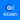# Solution: Find the equation whose roots are the reciprocals of the roots of the given equation

(Last Updated On: January 28, 2020)#### Problem Statement:

Find the equation whose roots are the reciprocals of the roots of the equation 2x^2-3x-5=0.

The equation whose roots are the reciprocals of the roots of the given equation is 5x^2 + 3x – 2 = 0.

#### Latest Problem Solving in Quadratic Equation, Binomial Theorem and Logarithms

More Questions in: Quadratic Equation, Binomial Theorem and Logarithms

#### Online Questions and Answers in Quadratic Equation, Binomial Theorem and Logarithms

Help Me Makes a Difference!

 P inoyBIX educates thousands of reviewers/students a day in preparation for their board examinations. Also provides professionals with materials for their lectures and practice exams. Help me go forward with the same spirit. “Will you make a small gift today via GCASH?”+63 966 459 6474 Option 1 : \$1 USD Option 2 : \$3 USD Option 3 : \$5 USD Option 4 : \$10 USD Option 5 : \$25 USD Option 6 : \$50 USD Option 7 : \$100 USD Option 8 : Other Amount© 2014 PinoyBIX Engineering. © 2019 All Rights Reserved | How to Donate? |